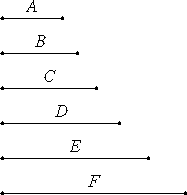# Proposition 10

If as many numbers as we please beginning from a unit are in continued proportion, and the number after the unit is not square, then neither is any other square except the third from the unit and all those which leave out one; and, if the number after the unit is not cubic, then neither is any other cubic except the fourth from the unit and all those which leave out two.

Let there be as many numbers as we please, A, B, C, D, E, and F, beginning from a unit and in continued proportion, and let A, the number after the unit, not be square.

I say that neither are any other square except the third from the unit and those which leave out one.IX.8

If possible, let C be square. But B is also square, therefore B and C have to one another the ratio which a square number has to a square number.

VIII.26 converse

And B is to C as A is to B, therefore A and B have to one another the ratio which a square number has to a square number, so that A and B are similar plane numbers.

And B is square, therefore A is also square, which is contrary to the hypothesis.

Therefore C is not square. Similarly we can prove that neither is any other of the numbers square except the third from the unit and those which leave out one.

Next, let A not be a cube.

I say that neither are any other cubes except the fourth from the unit and those which leave out two.

If possible, let D be a cube.

Now C is also a cube, for it is fourth from the unit. And C is to D as B is to C, therefore B has to C the ratio which a cube has to a cube. And C is a cube, therefore B is also a cube.

And since the unit is to A as A is to B, and the unit measures A according to the units in it, therefore A also measures B according to the units in itself. Therefore A multiplied by itself makes the cubic number B.

IX.6

But, if a number multiplied by itself makes cubic number, then it is itself a cube. Therefore A is also a cube, contrary to the hypothesis. Therefore D is not a cube. Similarly we can prove that neither is any other of the numbers a cube except the fourth from the unit and those which leave out two.

Therefore, if as many numbers as we please beginning from a unit are in continued proportion, and the number after the unit is not square, then neither is any other square except the third from the unit and all those which leave out one; and, if the number after the unit is not cubic, then neither is any other cubic except the fourth from the unit and all those which leave out two.

Q.E.D.

## Guide

This is a converse of the previous theorem.